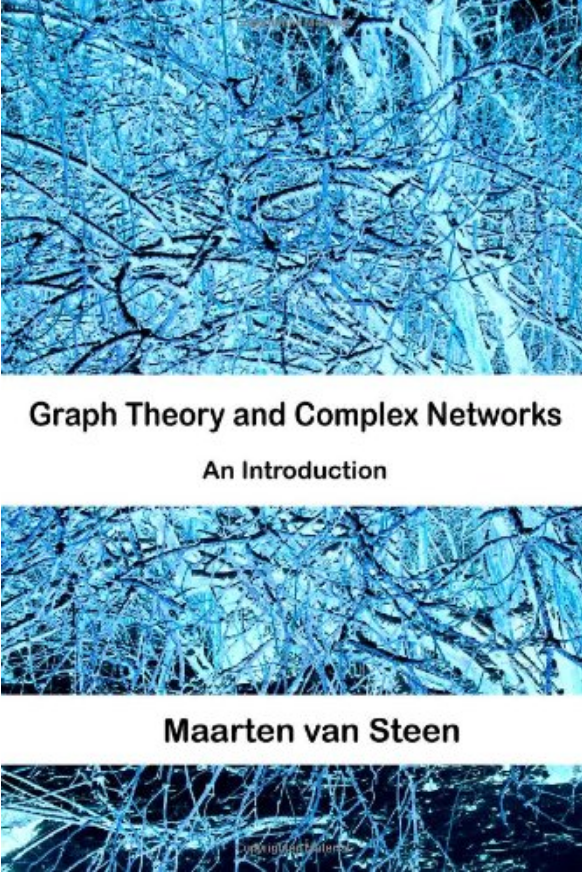# Graph Theory and Complex Networks## Goals

GTCN aims to explain the basics of graph theory that are needed at an introductory level for students in computer or information sciences. To motivate students and to show that even these basic notions can be extremely useful, the book also aims to provide an introduction to the modern field of network science.

I take the starting-point that mathematics for most students is unnecessarily intimidating. Explicit attention is paid in the first chapters to mathematical notations and proof techniques, emphasizing that the notations form the biggest obstacle, not the mathematical concepts themselves. Taking this approach has allowed me to gradually prepare students for using tools that are necessary to put graph theory to work: complex networks.

In the second part of the book the student learns about random networks, small worlds, the structure of the Internet and the Web, and social networks. Again, everything is discussed at an elementary level, but such that in the end students indeed have the feeling that they:

1. Have learned how to read and understand the basic mathematics related to graph theory
2. Understand how basic graph theory can be applied to optimization problems such as routing in communication networks